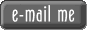VisitorsSuggestions
LAND MEASUREMENT CONVERSION GUIDE1 Acre = 43,560 square feet
1 Acre = 160 square rods
1 Acre = 1.1834 square arpents
1 Acre = 10 square chains
1 Acre = 160 square rods
1 Acre = 160 perches
1 Acre = 160 poles
1 Acre = .4047 hectare
1 Acre = 4047 square meters
1 Acre = is about 208 3/4 feet square
1 Acre Square = 5645.376 square varas
Arpen measurements vary by locality:
1 Arpent (in LA, MS, AL, FL) = .84625 of an acre
1 Arpent Square (in LA, MS, AL, FL) = 191.994 feet or 2.909 chains on each side
1 Arpent (AR and MO) = .8507 of an acre
1 Arpent Square (AR and MO) = 192.5 feet or 2.91667 chains on each side
1 Caballeria (Texas-Spanish) = 108 acres
1 Centimeter = .3937 inches
1 Centimeter = .032808 feet
1 Chain = 66 feet
1 Chain = 4 rods
1 Chain = 4 perches
1 Chain = 4 poles
1 Chain = 20.1168 meters
1 Foot = 12 inches
1 Foot = .36 varas
1 Furlong = 660 feet
1 Furlong = 40 rods
1 Foot = 0.3048006 meter
1 Hectare = 10,000 square meters
1 Hectare = 2.471 acres
1 Inch = .0254 meter
1 Kilometer = 3280.83 feet
1 Kilometer = .62 mile
1 Knot = 6080.2 feet
1 Labor (Texas-Spanish)= 1,000,000 square varas
1 Labor = 177.136 acres
1 League (Texas-Spanish) = 25,000,000 square varas
1 League = 4428.4 acres
1 Meter = 3.280833 feet
1 Meter = 39.37 inches
1 Meter Square = 10.764 square feet
1 Mile = 5,280 feet
1 Mile = 8 furlongs
1 Mile = 320 rods
1 Mile = 80 chains
1 Mile = 1.60935 kilometers
1 Mile = 320 perches
1 Mile = 320 poles
1 Mile = 1,609.2655 meters
1 Mile Square = a regular Section of land
1 Mile Square = 27,878,400 square feet
1 Mile Square = 640 acres
1 Mile Square = 259 hectares
1 Mile Square = 2.59 square hectares
1 Perch = 1 pole
1 Perch = 1 rod
1 Perch = 16.5 feet
1 Pole = 16.5 feet
1 Pole = 1 perch
1 Pole = 1 Rod
1 Rod = 1 pole
1 Rod = 1 perch
1 Rod = 16.5 feet
1 Section = 1 mile long, by 1 mile wide
1 Section = 640 acres
1 Sitio (Texas-Spanish)= 1 league
1 Township = 6 miles long, by 6 miles wide
1 Township = 36 sections
1 Township = 36 square miles
Vara Measurements differ by locality:
1 Vara (Texas-Spanish) = 33 1/3 inches
1 Vara (Southern Colorado) 32.993 inches
1 Vara (Florida) 33.372 inches
1 Yard = 36 inches
1 Yard = 3 feet
1 Yard Square = 9 square feet